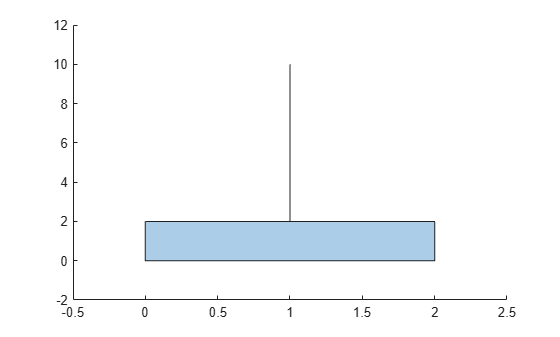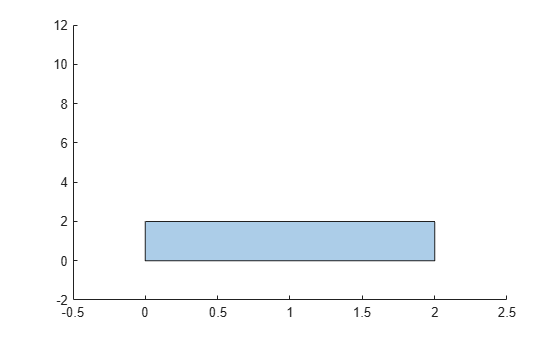# rmslivers

Remove `polyshape` boundary outliers

## Syntax

``polyout = rmslivers(polyin,tol)``

## Description

example

````polyout = rmslivers(polyin,tol)` returns a `polyshape` object made up of the boundaries of `polyin` with any outlier vertices removed. `tol` defines boundary outliers based on the ratio of relative distances between vertices.```

## Examples

collapse all

Remove an outlier boundary point from a polygon, eliminating the extraneous sliver or antenna effect.

```polyin = polyshape([0 0 2 2 1.0001 1 0.9999],[2 0 0 2 2 10 2]); plot(polyin) xlim([-0.5 2.5]) ylim([-2 12])``````polyout = rmslivers(polyin,0.001); plot(polyout) xlim([-0.5 2.5]) ylim([-2 12])```## Input Arguments

collapse all

Input `polyshape`, specified as a scalar, vector, matrix, or multidimensional array.

Data Types: `polyshape`

Outlier tolerance, specified as a scalar. When the input `polyshape` is an array, `rmslivers` removes outliers from each element of the array according to `tol`.

Data Types: `double` | `single` | `int8` | `int16` | `int32` | `int64` | `uint8` | `uint16` | `uint32` | `uint64`

collapse all

### Outlier Tolerance

The outlier tolerance specifies the allowable ratio between the relative distances of vertices in a polygon region. For example, in the following figure, if $\frac{a}{b}\le tol$ for a specified tolerance tol, then `rmslivers` does not remove the vertex v. If $\frac{a}{b}>tol$, then `rmslivers` removes v, eliminating the sliver.## Version History

Introduced in R2017b